# Basics¶

## Input-Output Methods¶

For taking input in python 2, we use raw_input().

$python >>>raw_input() this is taken as input 'this is taken as input'  In python3, there is no need for we use input(). $ python3
>>>input()
this is taken as input
'this is taken as input'

By default input is taken as string.

>>>n=input()
3
>>>print(type(n))
<class 'str'>

To convert the datatype, we need to typecast it separately.

Typecast: Converting the datatype of a variable from one to another.

>>>n=int(input())
3
>>>print(type(n))
<class 'int'>

In a similar way we can change the data type from int to float and many more.

• In all the above cases, if python 2 is used then, in place of input() use raw_input().

• For print statememt in python3, the synatx is print("Secure"). While in python 2, there is no need of parenthesis () but it won't show any error even if we use parenthesis (). It is print "Secure" or print ("Secure" ) .

## Operations¶

>>> a=5
>>> b=6
>>>a + b
11
>>>a - b
-1
>>>a * b
30
>>>a / b
0.8333333333333334
>>>a // b
0
>>>a % b
5
>>>a ** b
15625

• // shows the quotient.
• % show the remainder.
• ** is a to the power b or a raised to b.

In python 2 when '/ ' operation is used, the integer is printed while in case python 3 it will show value after decimal place also. So to obtain the float value in python 2 you need to specify at least one, either denominator or numerator as float.

$python >>> 3/2 1 >>>3.0/2 1.5  ## Comparison operators:¶ • a == b Equal to condition. • a < b Less than condition. • a > b Greater than condition. • a <= b Less than or equal to condition. • a >= b Greater than or equal to condition. • a != b Not equal to condition. ## Assignment Operator '='¶ a=b the value of b is assigned to a. ## Binary Operations¶ To understand this, use python built in function bin(n). It will show the binary form of number n. >>> bin(3) '0b11' >>> bin(2) '0b10'  #### a&b bitwise AND of a,b:¶ >>> 3&2 2  Each bit is taken taken and AND operation is performed. so the result we get is 0b010, which is 2.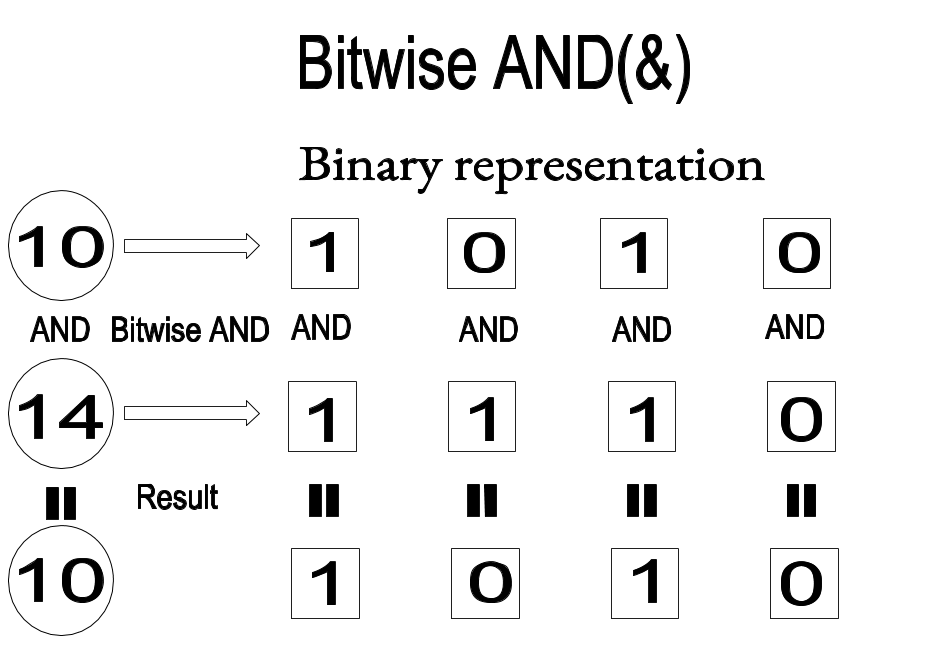#### a|b bitwise OR of a,b:¶ >>> 3|2 3  Each bit is taken and OR operation is performed. so the result we get is 0b11, which is 3.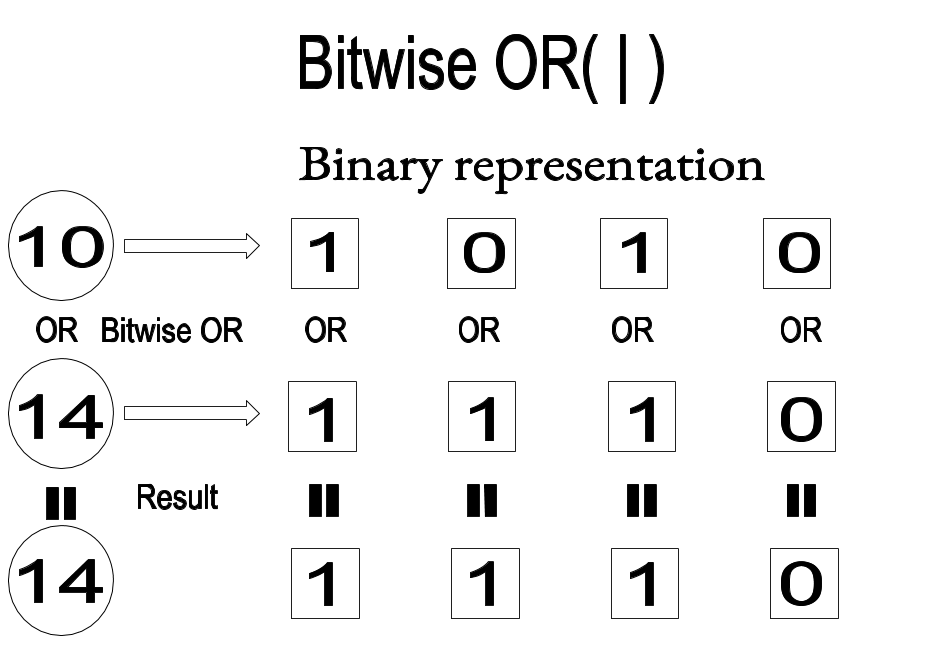#### a^b bitwise XOR of a,b:¶ >>> 3^2 1  Each bit is taken and XOR operation is performed. In XOR, if both bits are same then the result is 0, else 1. So 1^1 is 0 while 1^0 is 1. Hence, the result we get for 3^2 (0b11^0b10) is 0b001, which is 1.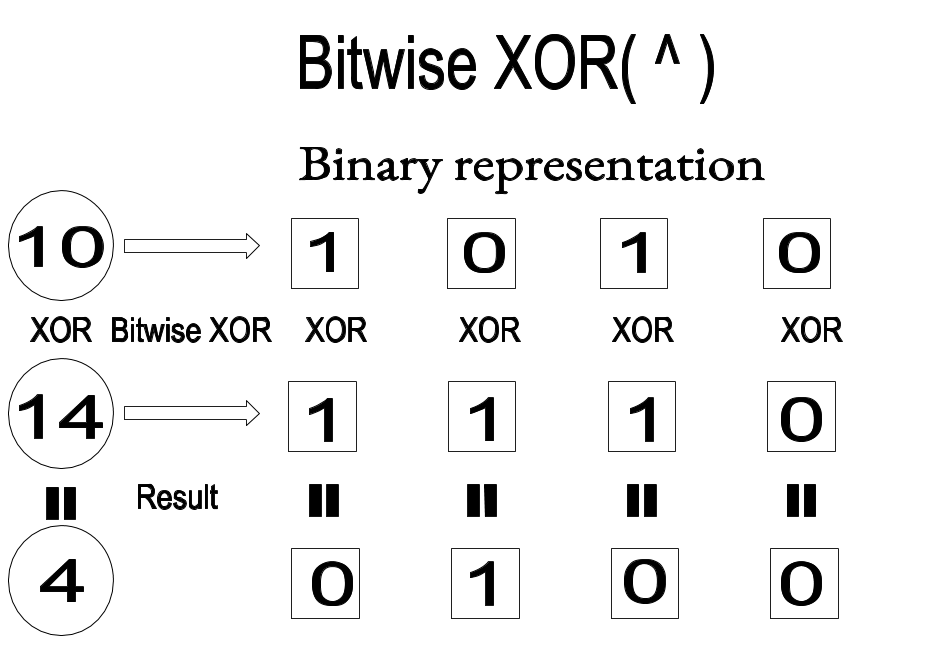### a<<b Left Shift¶ a<<b it will shift the bits of a in binary format to left, this shift is done b times: >>> 3<<2 12 >>>bin(12) 0b1100  Binary representation of 3 is 0b11, which is shifted twice to left. So the result is 0b1100 that is 12.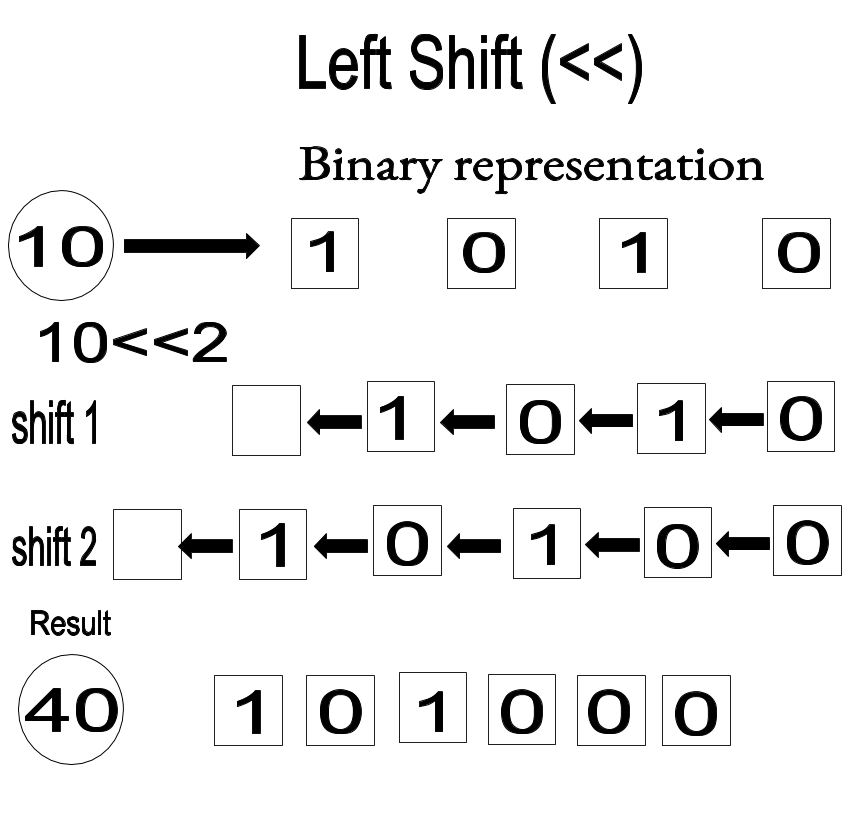### a>>b Right Shift¶ a>>b it will shift the bits of a in binary format to right, this shift is done b times: >>> 3>>1 1  Binary representation of 3 is 0b11, which is shifted once to right. So the result is 0b1 that is 1.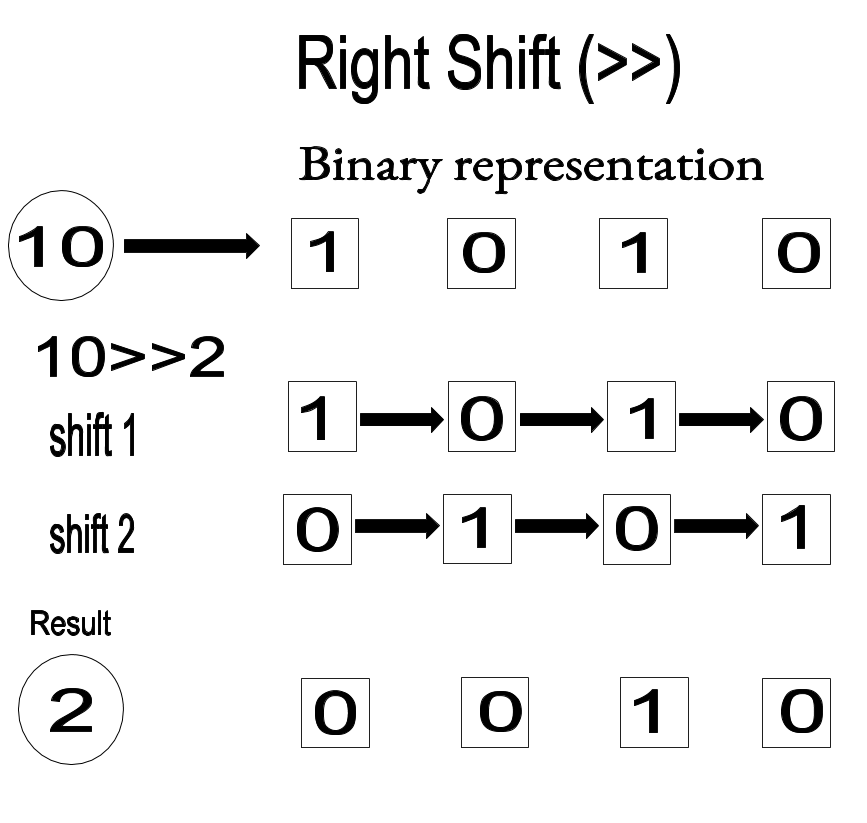Python can also understand logical operations when written in english : - and - or - not - in ## Conditional Statements¶ ### if statements¶ If condition statements are to be used when you have a set of statements which is to be executed when a particular condition is satisfied. For example if a person's age is above 18, he is eligible to vote. If not, he is not eligible to vote. Example 1 $ python3
>>>age=int(input())
32
>>>if(age>=18):
...    print(“you are eligible to vote”)
...else:
...    print(“you are not eligible to vote”)

Press enter button twice for output.

Output

you are eligible to vote
>>>

Example 2

$python >>>age=int(raw_input()) 32 >>>if age>=18: ... print “you are eligible to vote” ...else: ... print “you are not eligible to vote”  Press enter button twice for output. Output you are eligible to vote >>>  Example 3 If we have more than one condition to check we can use elif statements. $ python3
>>>age=int(input())
32
>>>if(age<=12):
...     print(“you are a kid”)
...elif(age>12 and age <= 19):
...     print (“you are a teenager”)
...elif(age >19 and age < 30 ):
...else:
print(“you are a senior citizen”)

Press enter button twice for output.

Output

you are a senior citizen
>>>

Example 4

$python >>>age=int(raw_input()) 32 >>>if age<=12 : ... print “you are a kid” ...elif age>12 and age <= 19 : ... print “you are a teenager” ...elif age >19 and age < 30 : ... print “you are an adult” ...else: print “you are a senior citizen”  Press enter button twice for output. Output you are a senior citizen >>>  ## Loops ### For loop It is used when you need to execute a set of statements n times. Syntax : sh for variable in range(start,end,incrementation): statements  If not mentioned then starting is taken as 0 and incrementation as 1 to the specified end point. This code is valid for both python 3 and python 2. Example 1 $ python
>>>for i in range(3):
...     print(“welcome to the world of security”)


Press enter button twice for output. Output

welcome to the world of security
welcome to the world of security
welcome to the world of security
>>>

Example 2

This code is valid for both python 3 and python 2.

$python3 >>>i = "teambi0s" >>>for i in c: ... print(i)  Press enter button twice for output. Output t e a m b i 0 s >>>  ### While loop¶ It is used to execute a set of statements until the condition is satisfied and hence the loop will end as the condition becomes false. Syntax : while(condition): statements  Example 1 This code is valid for both python 3 and python 2. $ python3
>>>flag=0
>>>while(flag!=3):
...     print(flag)
...     flag=flag+1

Press enter button twice for output.

Output

0
1
2
>>>


### Nested Loops:¶

Loop inside a loop is termed as nested.

This code is valid for both python 3 and python 2.

\$ python3
>>>for i in range (3):
...     for j in range (2):
...         print(“this is nested”)

Press enter button twice for output.

Output

this is nested
this is nested
this is nested
this is nested
this is nested
this is nested
>>>


It will print 6 times.